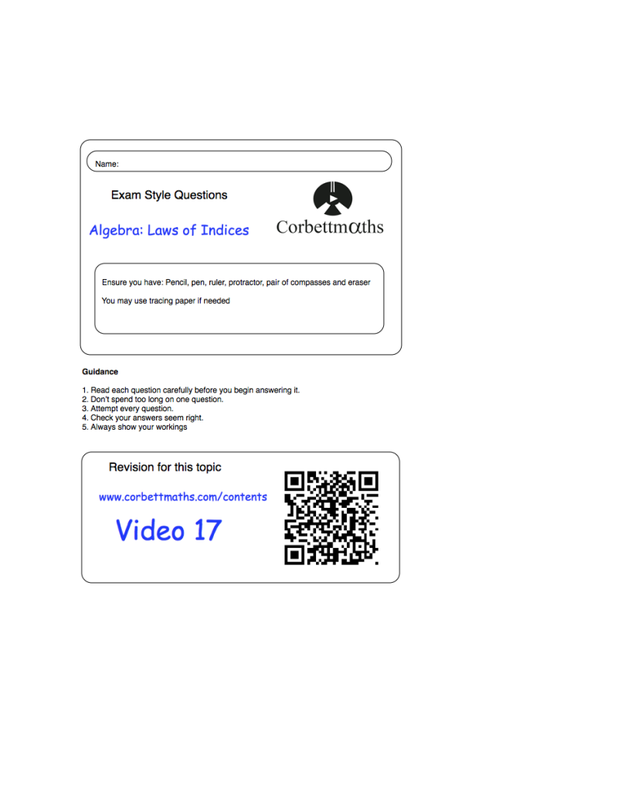GCSE Mathematics
04 Linear Equations And Inequalities Ppt For Teaching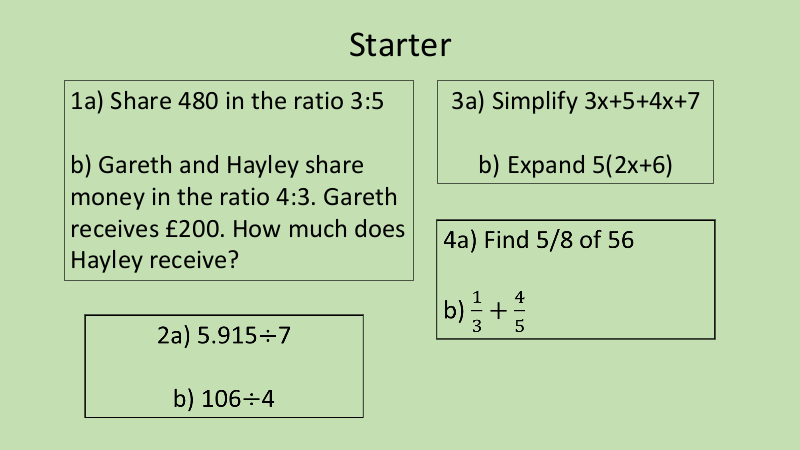Solving Equations - Cool math Pre-Algebra Help Lessons - Keep It Balanced
This prealgebra lesson explains why equations need to stay balanced as you solve them.
Algebra Formula powerpointSolving Equations Basics and rulesSimplifying Expressions and EquationsCommon Mistakes in Algebra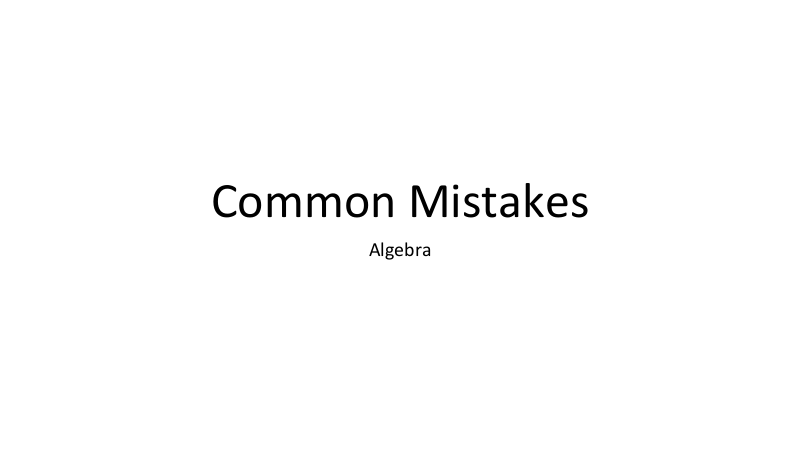Formulae worksheet questions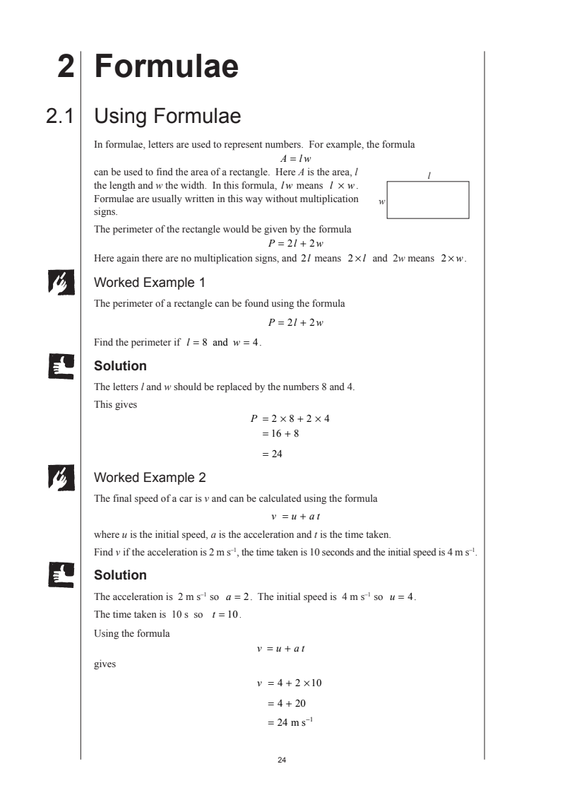Changing the Subject of a Formula Worksheet Corbett Maths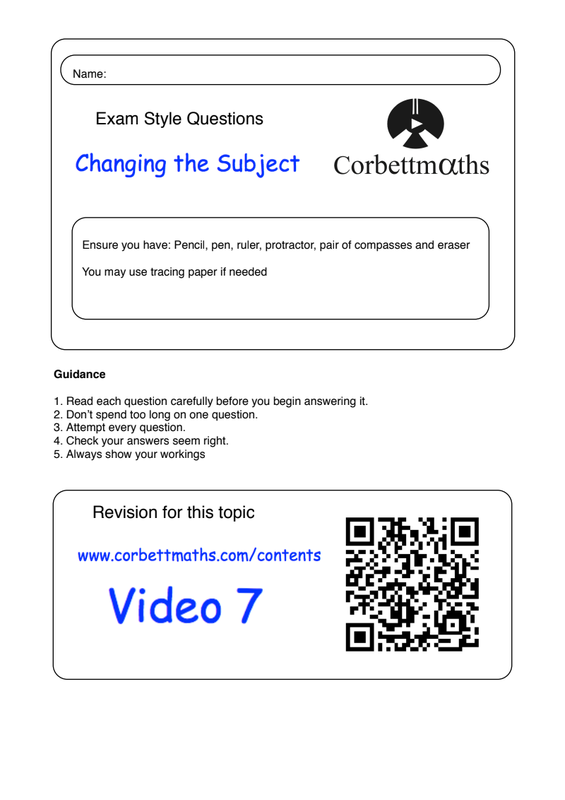Algebra Law of Indicies Worksheet ANSWERS